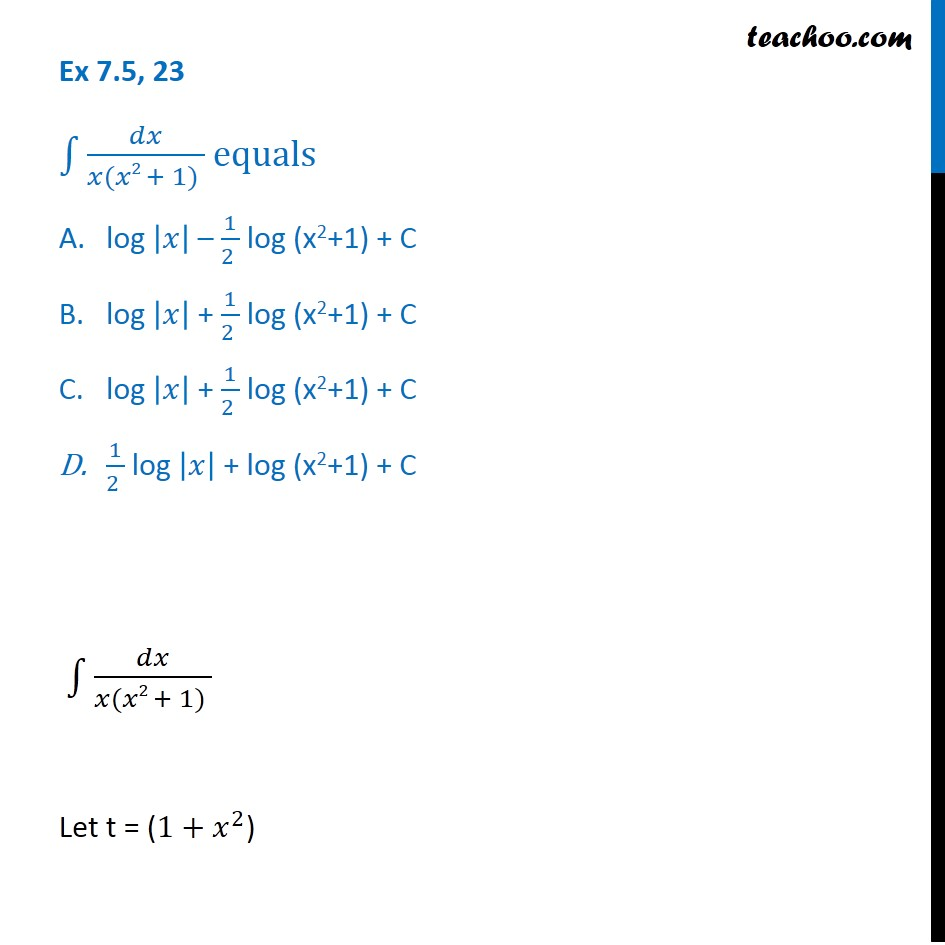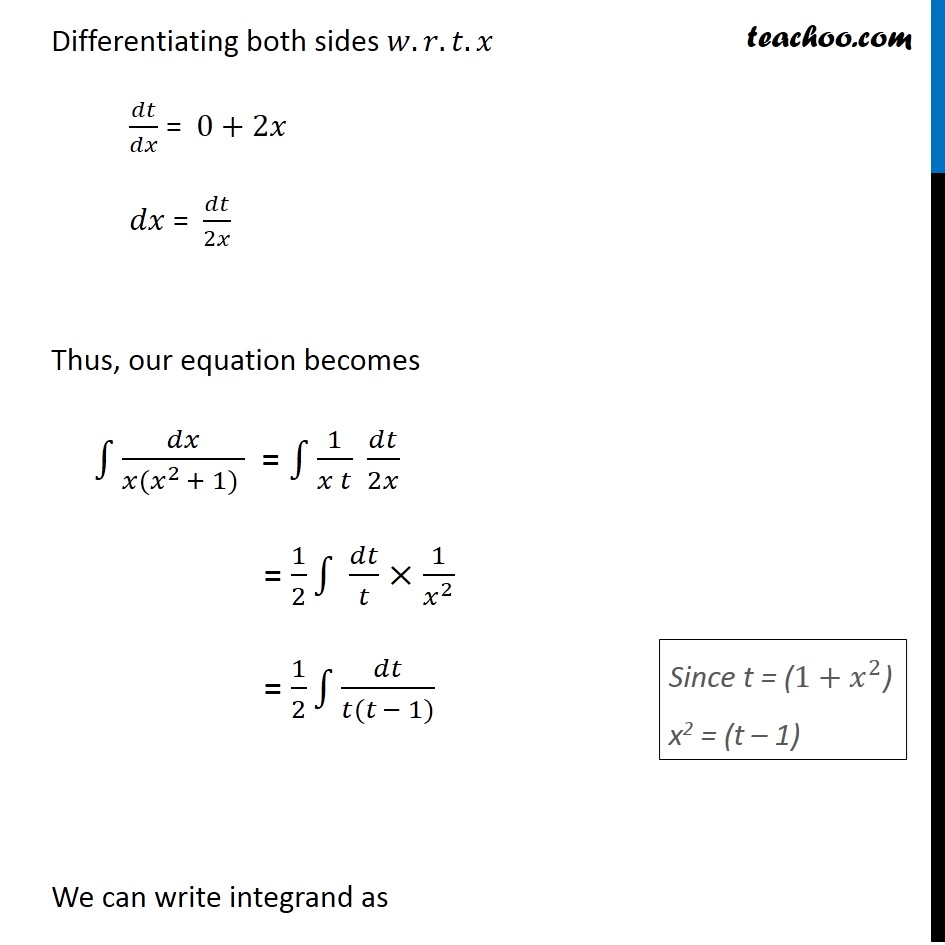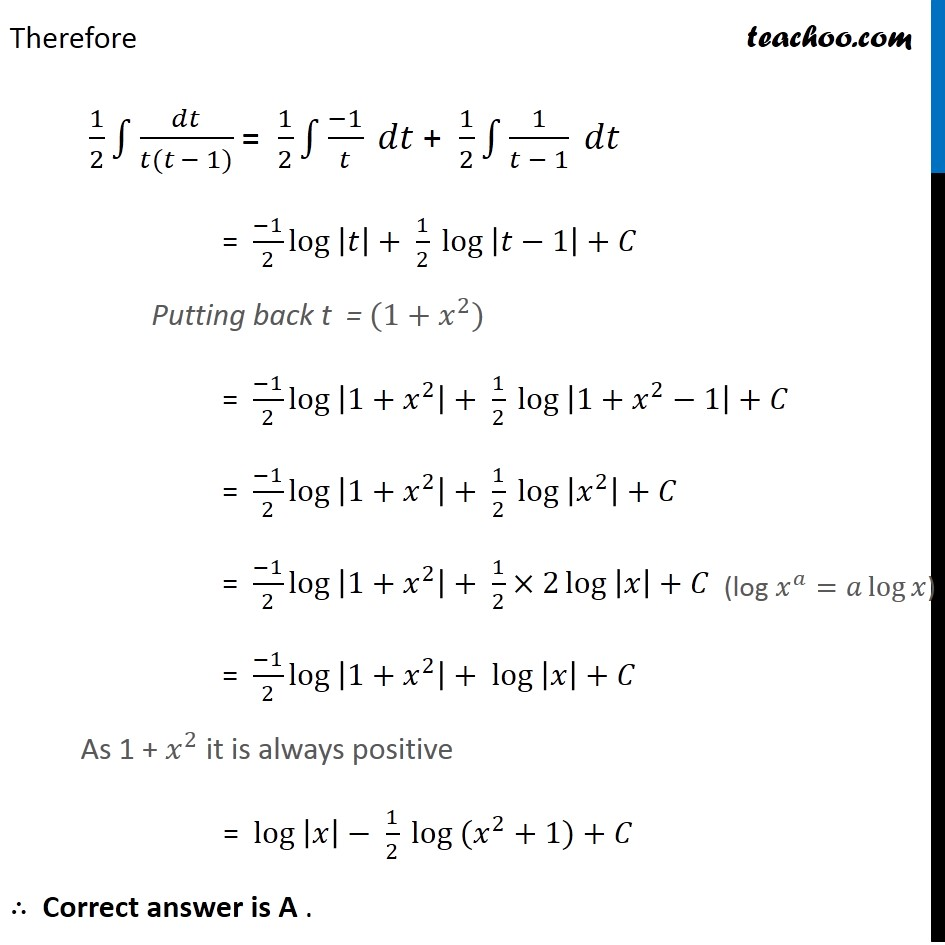Ex 7.5

Chapter 7 Class 12 Integrals
Serial order wiseLearn in your speed, with individual attention - Teachoo Maths 1-on-1 Class

### Transcript

Ex 7.5, 23 ∫1▒𝑑𝑥/(𝑥(𝑥2 + 1) ) equals log |𝑥| – 1/(2 ) log (x2+1) + C log |𝑥| + 1/(2 ) log (x2+1) + C log |𝑥| + 1/(2 ) log (x2+1) + C 1/(2 ) log |𝑥| + log (x2+1) + C ∫1▒𝑑𝑥/(𝑥(𝑥2 + 1) ) Let t = (1+𝑥^2) Differentiating both sides 𝑤.𝑟.𝑡.𝑥 𝑑𝑡/𝑑𝑥 = 0+2𝑥 𝑑𝑥 = 𝑑𝑡/2𝑥 Thus, our equation becomes ∫1▒𝑑𝑥/(𝑥(𝑥^2 + 1) ) = ∫1▒1/(𝑥 𝑡) 𝑑𝑡/2𝑥 = 1/2 ∫1▒〖 𝑑𝑡/𝑡×1/𝑥^2 〗 = 1/2 ∫1▒𝑑𝑡/(𝑡(𝑡 − 1)) We can write integrand as Since t = (1+𝑥^2) x2 = (t – 1) 1/(𝑡(𝑡 − 1)) = 𝐴/𝑡 + 𝐵/(𝑡 − 1) 1/(𝑡(𝑡 − 1)) = (𝐴(𝑡 − 1) + 𝐵𝑡)/(𝑡(𝑡 − 1)) Cancelling denominator 1 = 𝐴(𝑡−1)+𝐵𝑡 Putting t = 0 in (1) 1 = 𝐴(0−1)+𝐵×0 1 = −𝐴 𝐴 = −1 Putting t = 1 in (1) 1 = 𝐴(1−1)+𝐵(1) 1 = 𝐴×0+𝐵 1 = 𝐵 𝐵 = 1 Therefore 1/2 ∫1▒𝑑𝑡/(𝑡(𝑡 − 1)) = 1/2 ∫1▒〖(−1)/𝑡 〗 𝑑𝑡 + 1/2 ∫1▒〖1/(𝑡 − 1) 〗 𝑑𝑡 = (−1)/2 〖log 〗⁡|𝑡|+ 1/2 〖log 〗⁡|𝑡−1|+𝐶 Putting back t = (1+𝑥^2) = (−1)/2 〖log 〗⁡|1+𝑥^2 |+ 1/2 〖log 〗⁡|1+𝑥^2−1|+𝐶 = (−1)/2 〖log 〗⁡|1+𝑥^2 |+ 1/2 〖log 〗⁡|𝑥^2 |+𝐶 = (−1)/2 〖log 〗⁡|1+𝑥^2 |+ 1/2×2 〖log 〗⁡|𝑥|+𝐶 = (−1)/2 〖log 〗⁡|1+𝑥^2 |+ 〖log 〗⁡|𝑥|+𝐶 As 1 + 𝑥^2 it is always positive = 〖log 〗⁡|𝑥|− 1/2 〖log 〗⁡〖(𝑥^2+1)〗+𝐶 ∴ Correct answer is A . (log 𝑥^𝑎=𝑎 log⁡𝑥)